# Simple Laplace Transform Circuit Analysis ExamplesWe can use the Laplace transform to analyze an electric circuit. This is known as the Laplace transform circuit analysis, as the application of Laplace transform.

## Laplace Transform Circuit Analysis

Circuit analysis is again relatively easy to do when we are in the s-domain. We merely need to transform a complicated set of mathematical relationships in the time domain into the s-domain where we convert operators (derivatives and integrals) into simple multipliers of s and 1/s.

This now allows us to use algebra to set up and solve our circuit equations. The exciting thing about this is that all of the circuit theorems and relationships we developed for dc circuits are perfectly valid in the s-domain.

Remember, equivalent circuits, with capacitors and inductors, only exist in the s-domain; they cannot be transformed back into the time domain.

## Laplace Transform Circuit Analysis Examples

1. Consider the circuit in Figure.(1a). Find the value of the voltage across the capacitor assuming that the value of
vs(t) = 10u(t)
and assume that at t = 0, –1 A flows through the inductor and +5V  is across the capacitor.

Solution:
Figure.(1b) represents the entire circuit in the s-domain with the initial conditions incorporated. We now have a straightforward nodal analysis problem.

Since the value of V1 is also the value of the capacitor voltage in the time domain and is the only unknown node voltage, we only need to write one equation.

or

where v(0) = 5 V and i(0) = –1 A. Simplifying we get

Taking the inverse Laplace transform yields

2. For the circuit shown in Figure.(1), and the initial conditions used in  Example 1, use superposition to find the value of the capacitor voltage.

Solution:
In as much as the circuit in the s-domain actually has three independent sources, we can look at the solution one source at a time. Figure.(2) presents the circuits in the s-domain considering one source at a time.

We now have three nodal analysis problems. First, let us solve for the capacitor voltage in the circuit shown in Figure.(2a).or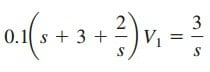Simplifying we get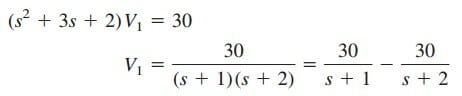or

For Figure.(2b) we get,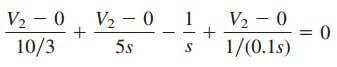or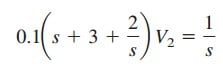Taking the inverse Laplace transform, we get

For Figure.(2c),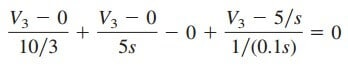or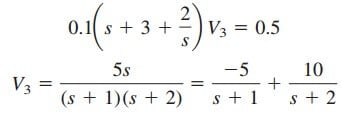This leads to

Now, all we need to do is to add Equations.(2.1), (2.2), and (2.3):or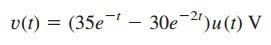which agrees with our answer in Example 1.

3. Assume that there is no initial energy stored in the circuit of Figure.(3) at t = 0 and that is = 10u(t) A.
(a) Find Vo(s) using Thevenin’s theorem.
(b) Apply the initial- and final-value theorems to find vo(0+) and vo(∞).
(c) Determine vo(t).

Solution:
Because there is no initial energy stored in the circuit, we assume that the initial inductor current and initial capacitor voltage are zero at t = 0.

(a) To find the Thevenin equivalent circuit, we remove the 5-Ω the resistor and then find Voc (VTh) and Isc. To find VTh, we use the Laplace transformed circuit in Figure.(4a).

Since Ix = 0, the dependent voltage source contributes nothing, so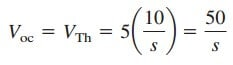To find ZTh, we consider the circuit in Figure.(4b), where we first find  Isc. We can use nodal analysis to solve for V1 which then leads to
Isc (Isc = Ix = V1∕2s).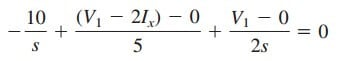along withHence,and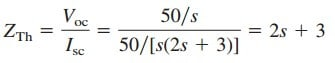The given circuit is replaced by its Thevenin equivalent at terminals
ab as shown in Figure.(5).

From Figure.(5),(b) Using the initial-value theorem we findUsing the final-value theorem we find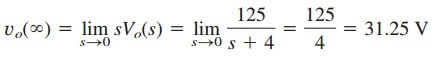(c) By partial fraction,Taking the inverse Laplace transform givesNotice that the values of vo(0) and vo(∞) obtained in part (b) are confirmed.